## 7595 Reputation

10 years, 257 days

## why Maple remember the sorted polynomial...

Maple 2023

Another very strange behavior.

res:= x^2 + x + 1 + 1/3*x^3 + 1/12*x^4 + 1/60*x^5 + 1/360*x^6 + 1/2520*x^7;
sort(res);

#and now
res

Without trying it, what do you expect the output of the last line above to be?  The same as the first one, right?  But that is not what Maple gives. Maple now changes res to always be sorted, even though I have not reassigned it.

Does this mean if one calls sort(), then all the variables that contains polynomials will also be sorted automatically?

Unable to upload worksheet. So here is screen shot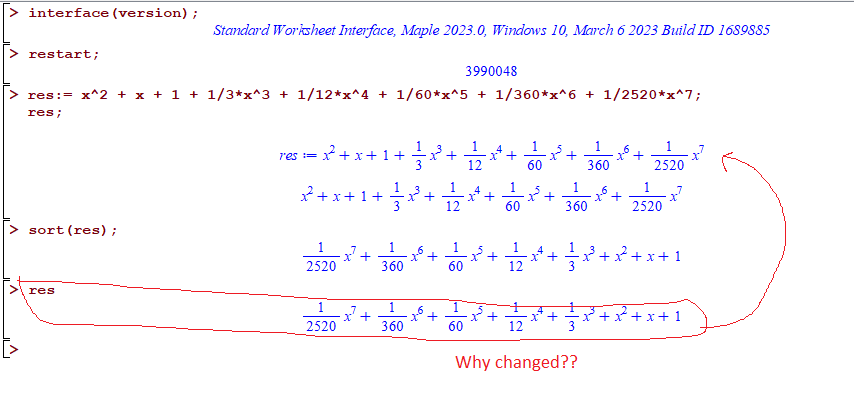## why this limitation in the limit() comma...

Maple 2023

These give errors

limit(y(0),y(0)=1)

limit(y(x),y(x)=1)



Error, (in limit) limit variable previously assigned, 2nd argument evaluates to y(0) = 1

One has to write

limit(y,y=1)


But why? Is this because y(x) is not considered "algebraic" ?

Mathematica has no problem with either one of these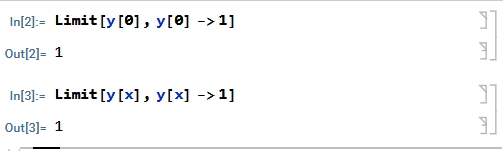I found this when I was trying to find the limit at initial conditions, when x=0 and y(0)=1 and wanted to take the limit on both x->0 and y(0)->1

I can easily code around this, by renaming y(0) to say Y0 and then the limit will work.

It just seems to be unnecessary restriction of the limit() command parsing ability.

What is the logic behind not allowing one to write limit(y(x),y(x)=1) ?

## why Maple's worksheet content disappears...

Maple 2023

I found a new problem in Maple 2023 on windows 10.  But it is more of an annoyonus. This is what happens:

I can have a worksheet open and running somecode with lots of output generated on the screen. Everything displays fine.

Now I go and open a new application not related to Maple at all, for example if I open the task manager, this causes the Maple screen to immediately go all BLANK ! Yes, all the display is gone. I have no idea why opening a new application in new window could cause this.

Everything disappears from the worksheet except for the worksheet frame. All the menus are gone also.

But resizing the worksheet window makes everything to appear agin due to the repaint process, But I need to resize the worksheet few times to get everything to repaint fully.

So the content is still there, but it just do not show/repaint.

Note that Maple does NOT freeze or anything, it is still running and the content is there, it is just that the content becomes not visible due to opening a new application window on the side.

I have never seen anything like this in any other application on windows. I also never had this problem on same PC with Maple 2022.2.

Anyone else noticed this problem?  This is reproducible all the time. Sometimes the content will display again on its own after short amount of time, but will be missing many menu items and missing side content and so on so I have to resize the windows to make repaint eveything again.

May be it is a Java problem. I do not know.

I have the same system as described in this post

I will make a movie to show this very soon but I am waiting for something to finish running before I do this so I can save all my work.

Windows 10 pro, running Maple 2023 with two monitors.

Here is a small movie. I made very small example which prints number on the screen in a loop. I added a Sleep in between so it does not take over the CPU just for illustration. In my real code there is no such Sleep command.

In this movie, we see that when I open the task manage window how the display in the worksheet is gone. In this example it comes back after few seconds but all the other items in the worksheet (menus etc..) are still missing. Resizing the windows makes them appear again. In my actual code, due to the larger content, the whole worksheet remain not visible for much longer each time (I waited 5 minutes and more for it to repaint), but still had to resize it to make everything visible as in this example.

Can someone else reproduce this?

for n from 1 to 1000000 do
print("n=",n);
od;## How to obtain degree of x to the power w...

Maple 2023

I am porting some code I have in Mathematica to Maple.

Mathematica has command Exponent which returns largest power on x, even if the power is not integer.

It is defined as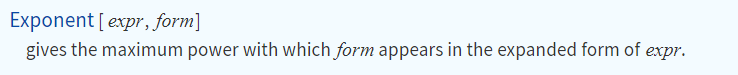Maple's degree command does not allow this and returns FAIL (since it is meant to work only on polynomial, I understand that).

Is there a different command I could use which does the same as Exponent? Otherwise I have to parse it manually. The expressions will all be univariant polynomial in x, but the power on x can contain a symbol and numbers. The expression can be more complicated, but it will always have just one x in it, and I just want to extract the power in that x inside the expression.

Here are examples

degree(x^2,x);
degree(x^(n-1),x);
degree(3*x^(n+2),x);


In the above all fail except the first one which returns 2. For reference here is the result from Mathematica's Exponent command

Exponent[x^2, x]
Exponent[x^(n - 1), x]
Exponent[3*x^(n + 2), x]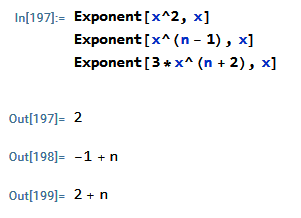I googled and could not find anything so far. I looked at PolynomialTools but do not see such command in it.

On possibility to do this manually is

get_exponent:=proc(expr,x::symbol)
local z:=indets(expr,identical(x)^anything);
if nops(z)<>0 then
op(2,z);
else
1;
fi;
end proc;


And now

get_exponent(x^2,x);
get_exponent(x^(n-1),x);
get_exponent(3*x^(n+2),x);


2

n-1

n+2

Eitherway, if there is a builtin command in Maple, it will be better to use than my code which might still fail for some cases I did not think of.

Maple 2023

## problem translating string to latex. Usi...

Maple 2023

The standard way of writing quotes and double quotes in LaTeX is with  and ''

So a string should be translated to Latex using the_string' '  and not the_string"  which is what Maple does.

see for example this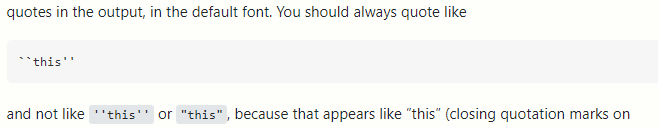For example, Maple translates  "regular" to

"\text{regular\"}"

It should be

"\text{regular' '}"

i.e. instead of closing it on the RIGHT with string quote  \"  it should close it with two upticks, like this ' '

Hard to see the differene here, so here is screen shot

s:="regular";
latex(s,output=string);
lprint(%)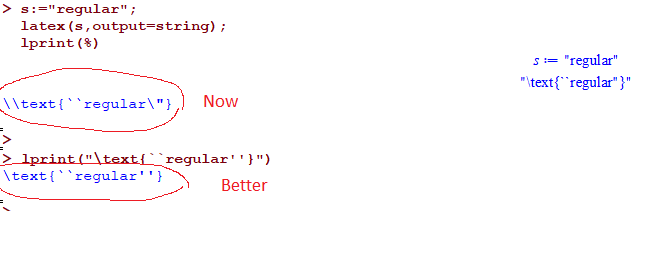The difference will show up depending on what font one is using. Here is an example below where when using different font, the effect is now visible.

\documentclass[12pt]{book}
\usepackage{amsmath}

\usepackage[T1]{fontenc}
\usepackage{mlmodern}

\begin{document}

\text{regular"}

\text{regular''}

\end{document}

The first one above is what Maple generates, the second one is what it should have been. Compiling with luatex gives this pdf, You can see clearly the difference now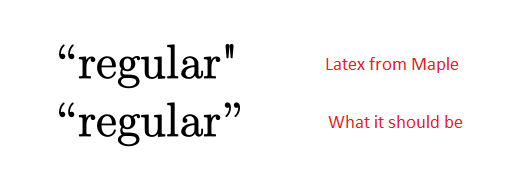It is important to note that this behavior shows depending on font used. For example, this code

\documentclass[12pt]{book}
\usepackage{amsmath}
\begin{document}

\text{regular"}

\text{regular''}

\end{document}


When compiled givesSo to be safe, it is better to always use the standard   ....' '   and not  .... \" because it then works the same for different fonts being used.

So, would it be possible to make Maple generate quoted string using the standard latex by closing it with ' ' instead of \"  ?

 1 2 3 4 5 6 7Last Page 1 of 147
﻿